# Laplace Transform Network Synthesis ExamplesLaplace transform network synthesis may be regarded as the process of obtaining an appropriate network to represent a given transfer function. Network synthesis is easier in the s-domain than in the time domain.

## Laplace Transform Network Synthesis

In network analysis, we find the transfer function of a given network. In network synthesis, we reverse the approach: Given a transfer function, we are required to find a suitable network.

Network synthesis is finding a network that represents a given transfer function.

Keep in mind that in synthesis, there may be many different answers—or possibly no answers—because there are many circuits that can be used to represent the same transfer function; in network analysis, there is only one answer.

Network synthesis is an exciting field of prime engineering importance. Being able to look at a transfer function and come up with the type of circuit it represents is a great asset to a circuit designer. Although network synthesis constitutes a whole course by itself and requires some experience, the following examples are meant to stimulate your appetite.

## Laplace Transform Network Synthesis Examples

1. Given the transfer functionrealize the function using the circuit in Figure.(1a).
(a) Select R = 5 Ω, and find L and C.
(b) Select R = 1 Ω, and find L and C.

Solution:
1. Define. The problem is clearly and completely defined. This problem is what we call a synthesis problem: Given a transfer function, synthesize a circuit that produces the given transfer function.

However, to keep the problem more manageable, we give a circuit that produces the desired transfer function. Had one of the variables, R in this case, not been given a value, then the problem would have had an infinite number of answers.

An open-ended problem of this kind would require some additional
assumptions that would have narrowed the set of solutions.

2. Present. A transfer function of the voltage out versus the voltage in is equal to 10/(s2 + 3s + 10). A circuit, Figure.(1), is also given that should be able to produce the required transfer function.

Two different values of R, 5 and 1 Ω, are to be used to calculate the values of L and  C that produce the given transfer function.

3. Alternative. All solution paths involve determining the transfer function of Figure.(1) and then matching the various terms of the transfer function. Two approaches would be to use mesh analysis or nodal analysis. Because we are looking for a ratio of voltages, the nodal analysis makes the most sense.

4. Attempt. Using nodal analysis leads to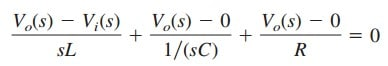Now multiply through by sLR: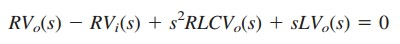Collecting terms we getorMatching the two transfer functions produces two equations with
three unknowns.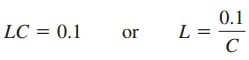and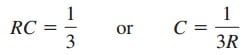We have a constraint equation, R = 5 Ω for (a) and = 1 Ω for (b).
(a) C = 1/(3 × 5) = 66.67 mF and L = 1.5 H
(b) C = 1/(3 × 1) = 333.3 mF and L = 300 mH

5. Evaluate. There are different ways of checking the answer. Solving for the transfer function by using mesh analysis seems the most straightforward and the approach we could use here.

However, it should be pointed out that this is mathematically more complex and will take longer than the original nodal analysis approach. Other approaches also exist.

We can assume an input for vi(t), vi(t) = u(t) V
and, using either nodal analysis or mesh analysis, see if we get the same answer we would get with just using the transfer function.

That is the approach we will try using mesh analysis.
Let vi(t) = u(t) V or Vi(s) = 1/s. This will produce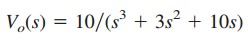Based on Figure 1, mesh analysis leads to

(a) For loop 1,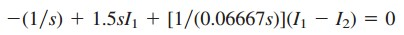orFor loop 2,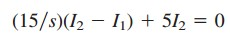orSubstituting into the first equation we get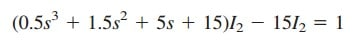orbut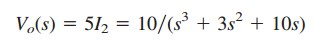and the answer checks.

(b) For loop 1,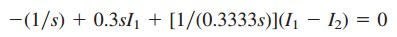orFor loop 2,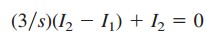orSubstituting into the first equation we getorbut Vo(s) = 1 × I2 = 10/(s3 + 3s2 + 10s)

6. Satisfactory? We have clearly identified values of L and C for each of the conditions. In addition, we have carefully checked the answers to see if they are correct. The problem has been adequately solved. The results can now be presented as a solution to the problem.

2. Synthesize the function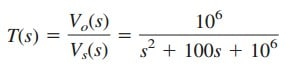using the topology in Figure.(2).

Solution:
We apply nodal analysis to nodes 1 and 2. At node 1,

At node 2,

But V2 = Vo, so Equation.(2.1) becomes

and Equation.(2.2) becomes

Substituting Equation.(2.4) into Equation.(2.3) givesor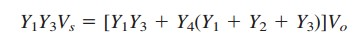Thus,

To synthesize the given transfer function T(s), compare it with the one in Equation.(2.5). Notice two things:
(1)Y1Y3 must not involve because the numerator of T(s) is constant;
(2) the given transfer function is second-order, which implies that we must have two capacitors.

Therefore, we must make Y1 and Y3 resistive, while Y2 and Y4 are capacitive. So we select

Substituting Equation.(2.6) into Equation.(2.5) gives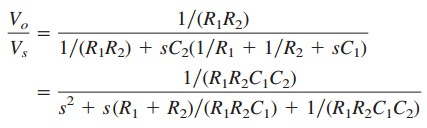Comparing this with the given transfer function T(s), we notice thatIf we select R1 = R2 = 10 kΩ, then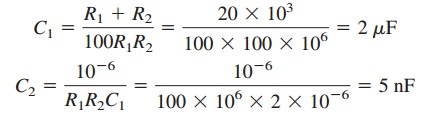Thus, the given transfer function is realized using the circuit shown in Figure.(3).